# Horizontal Line

A horizontal line is a straight line that goes from left to right or right to left. In coordinate geometry, a line is said to be horizontal if two points on the line have the same Y- coordinate points. It comes from the term “horizon”. It means that the horizontal lines are always parallel to the horizon or the x-axis. The graph of the horizontal line can be plotted in the cartesian plane.

## Horizontal Line Definition

The meaning horizontal line is a straight line that is mapped from left to right and it is parallel to the X-axis in the plane coordinate system. In other words, the straight line that does not make any intercept on the X-axis and it can have an intercept on Y-axis is called horizontal line. This means that the line that does not touch any point on the X-axis. A vertical line is always perpendicular to the horizontal line.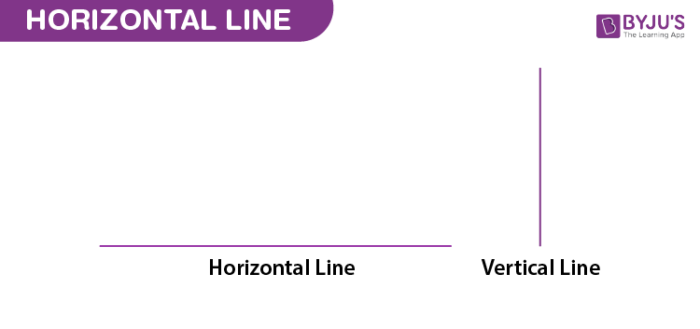## Horizontal Line Equation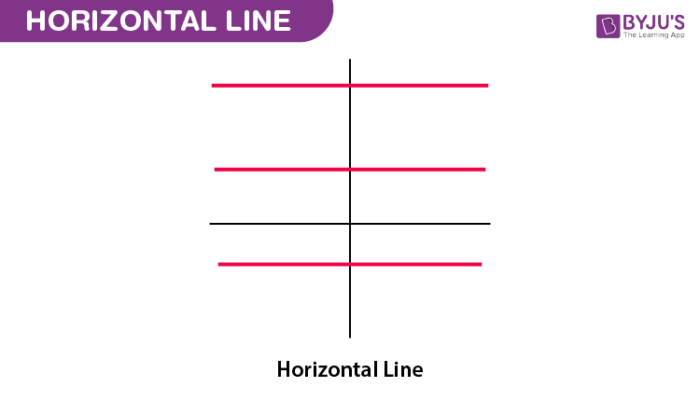The equation always takes the form:

 y = k

where k is the y-intercept.

It is observed that the horizontal lines have the same slope, so the slope of the horizontal line is always zero.

Examples of horizontal line equations are:

• y = 2
• y = -1
• y = -3
• y = 5
• y = 7

### Slope of Horizontal line

If we compare, the given equation, with the equation of line in the slope-intercept form, we get;

y = mx + c and y = k

m = 0

Therefore, the slope of the horizontal line is always equal to zero.

## How to Draw Horizontal Line?

Construction of horizontal line in XY coordinate is a simple method.

• To draw a horizontal line, we need to know the dimensions of point (i.e.,x and y value)
• Now, we can locate the point in the XY plane
• Taking the point (x,y) as the reference point, we need to draw a line parallel to the x-axis
• Thus, a horizontal line is drawn

Let us see some examples here.

Example 1: Draw the horizontal line y = 3.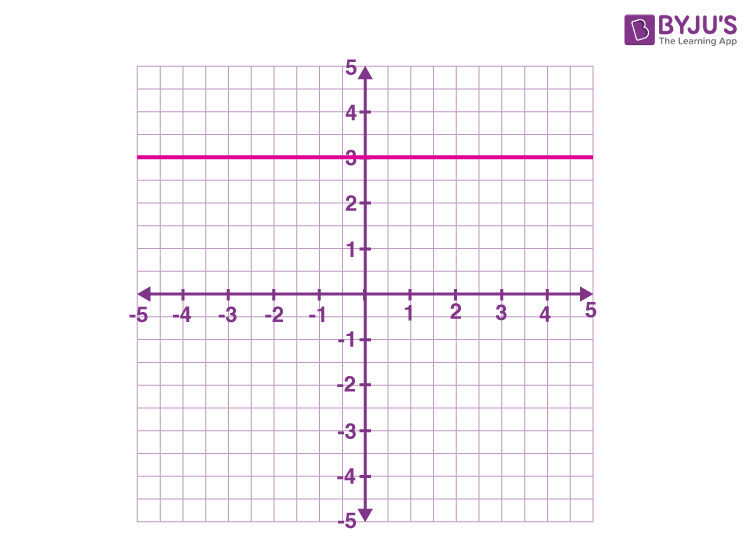Example 2: Draw the horizontal line y = -2.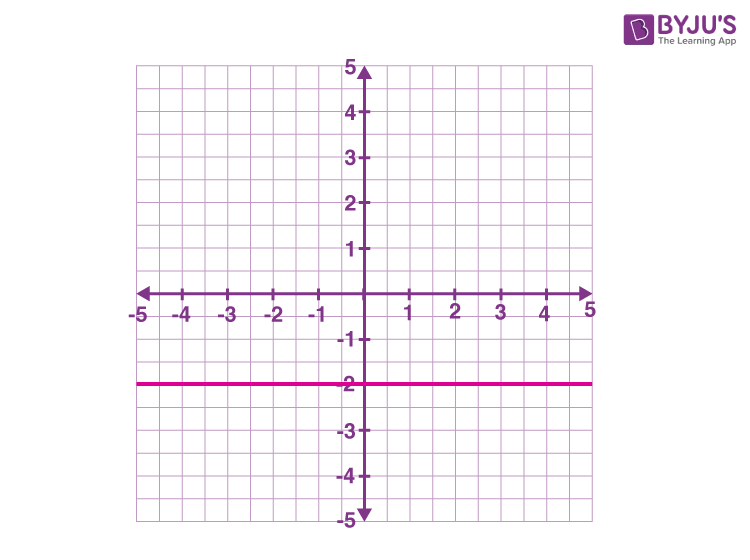## Horizontal Line Symmetry

The line of symmetry can be either horizontal, vertical or diagonal. If the line of symmetry is parallel to the horizontal plane, then it is known as the horizontal line of symmetry. We can also find the line of symmetry in shapes also. This means that the line divides the shapes into two parts as mirror images. Some of the examples of symmetry are given here.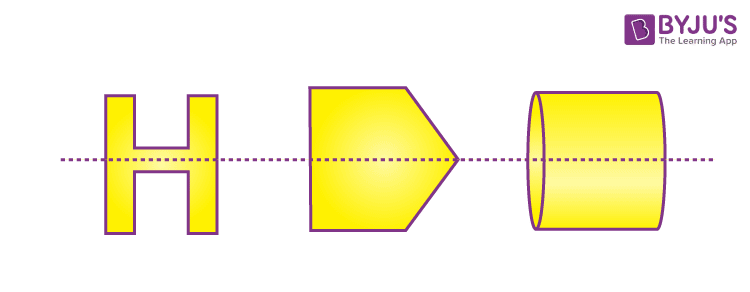## Horizontal Line Test

The test is used to find whether the function is one-to-one. (i.e., injective). For a given function, we can decide whether the function is injective or not, by looking at the horizontal lines that intersect the functional graph. This means that if the line that cuts the graph in more than one point, is not a one-to-one function. And also, this test is performed to find whether the function is bijective (one-to-one correspondence) or subjective (onto function).

## Horizontal Line Segment

It is defined as the part of the horizontal line that is limited by the two definite points. Those two points are called endpoints.

## Horizontal and Vertical Lines

Let us list the difference between horizontal and vertical lines here.

 Horizontal line Vertical line Parallel to horizon Perpendicular to horizontal lines It goes left to right or right to left It goes up to down or down to up Example: y = 3 is horizontal line equation Example: x = -2 is vertical line equation

The similarity between horizontal and vertical lines is both will have a one-variable equation.

## Solved Examples

Q.1: Determine the horizontal line equation, whose y-intercept is (0, 2)

Solution:

Given:

Y-intercept = (0, 2)

We know that,

The general equation of the horizontal line is y=k

Here, “k” is the y-intercept. So the value of k = 2

Therefore, the equation of the horizontal line is y = 2.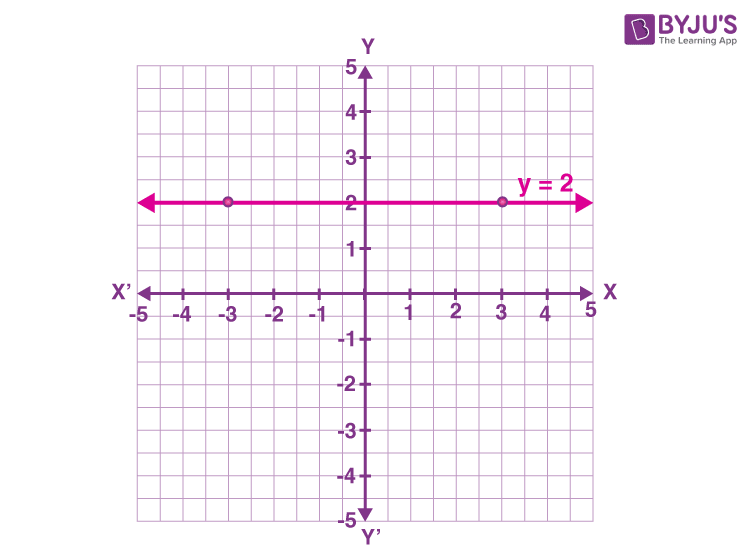Q.2: Determine the equation of the horizontal line given in the figure.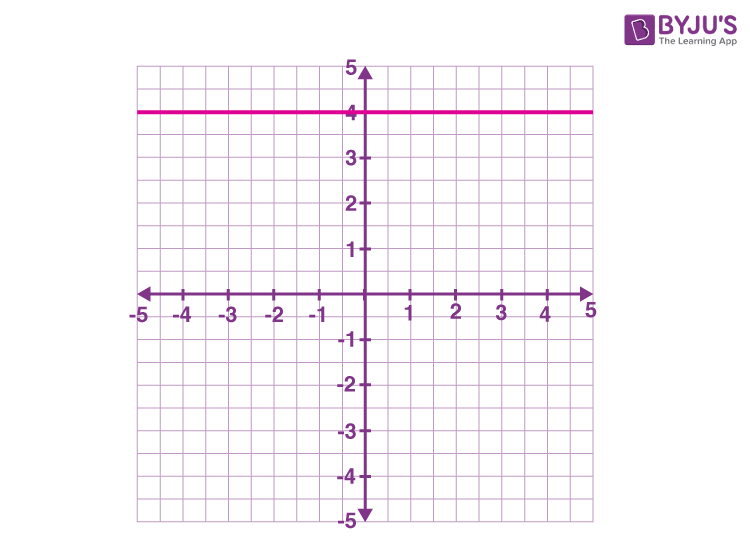Solution: We can see in the given figure that, the horizontal line passes through the point (0,4).

Hence, the equation of the line is y = 4.

## Practice Questions

1. Draw a line parallel to the x-axis and passing through the point (2,3)
2. Draw a horizontal line having the equation y = -3.5.
3. If a horizontal line passes through the point (1,-5), what is the equation of the line?

Visit BYJU’S – The Learning App for more maths-related articles in detail and also watch engaging videos to learn the concepts soon.

 Related Articles Line Distance Between Two Lines Intersecting and Non-Intersecting Lines Line of Symmetry

## Frequently Asked Questions – FAQs

Q1

### What is a horizontal line?

A horizontal line, in a coordinate plane, is a line that parallels the x-axis.
Q2

### What is the equation of a horizontal line?

Equation of the horizontal line is given by y = k, where k is any value on the y-axis.
Q3

### What is the slope of a horizontal line?

The slope of horizontal line is always equal to zero.
Q4

### If a line has an equation y = 3, then is it a horizontal or vertical line?

y = 3 is a horizontal line equation. The line will pass through the point y = 3 at y-axis and will be parallel to the x-axis.
Q5

### What is the example of vertical line equation?

A vertical line is a line that passes through x-axis and is parallel to the y-axis. Example of vertical line is x = -4.